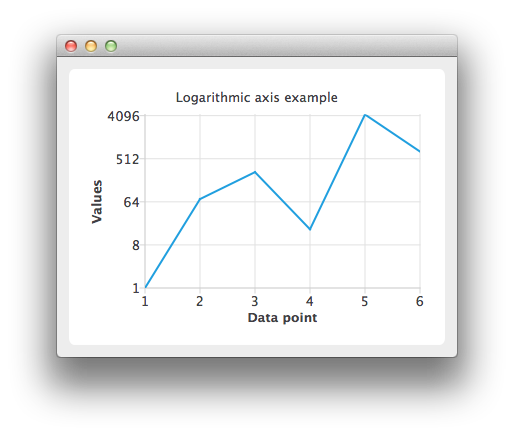# Logarithmic Axis Example

The example shows how to use QLogValueAxis.## Running the Example

To run the example from Qt Creator, open the Welcome mode and select the example from Examples. For more information, visit Building and Running an Example.

## Using Logarithmic Value Axes

Create a QLineSeries instance and add some data to it.

```QLineSeries *series = new QLineSeries();
*series << QPointF(1.0, 1.0) << QPointF(2.0, 73.0) << QPointF(3.0, 268.0) << QPointF(4.0, 17.0)
<< QPointF(5.0, 4325.0) << QPointF(6.0, 723.0);```

To present the data on the chart we need a QChart instance. Add the series to it, hide the legend and set the title of the chart.

```QChart *chart = new QChart();
chart->legend()->hide();
chart->setTitle("Logarithmic axis example");```

Create the axes. Add them to the chart and attach to the series.

```QValueAxis *axisX = new QValueAxis();
axisX->setTitleText("Data point");
axisX->setLabelFormat("%i");
axisX->setTickCount(series->count());
series->attachAxis(axisX);

QLogValueAxis *axisY = new QLogValueAxis();
axisY->setTitleText("Values");
axisY->setLabelFormat("%g");
axisY->setBase(8.0);
axisY->setMinorTickCount(-1);
series->attachAxis(axisY);```

Then create a QChartView object with QChart as a parameter. Enable antialiasing to have the rendered line look nicer.

```QChartView *chartView = new QChartView(chart);
chartView->setRenderHint(QPainter::Antialiasing);```

The chart is ready to be shown.

```QMainWindow window;
window.setCentralWidget(chartView);
window.resize(800, 600);
window.show();```

Files: